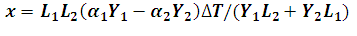# Displacement of joint of rod fixed at both ends due to change in temperature Calculator

This Calctown Calculator calculates the displacement of joint of a rod fixed at both ends due to change in temperature.

K-1
K-1
m
m
Pa
Pa
K

#### Result

mClick here to view image

where

x = displacement of joint

ΔT = change in temperature

α1, α2 are coefficients of linear thermal expansion of the strips with α2 > α1.

L1, L2 are the respective lengths of the strips

Y1, Y2 are the respective young's modulii of the strips